## CVR using hypercapnia

The test data for this tutorial consists of a pre-processed BOLD dataset acquired using a 3 tesla Philips MRI system with corresponding end-tidal CO2 and O2 respiratory traces measured using a RespirAct computer controlled gas delivery system (https://thornhillmedical.com/research/respiract-ra-mr/). The respiratory protocol consisted of a baseline period followed by a longer hypercapnic block and then two shorter hypercapnic blocks. Due to hosting constraints, the data files are separated into 5 parts. For part 2-5, remove the .zip extension (DO NOT delete the .zip extension for the first file) and then simply unzip the test data file and update the data path (/Data) pointing to the image files along with the sequence path (/Data/traces) in the Matlab live script (.mlx) file.

TUTORIAL 1: BOLD-CVR based on computer controlled CO2 stimulus

# TUTORIAL 1: BOLD-CVR based on computer controlled CO2 stimulus

This tutorial comprises a template data analysis script that utilizes native matlab functions as well as functions from the seeVR toolbox. If you use any part of this process or functions from this toolbox in your work please cite the following article:
... and toolbox
Bhogal AA, (2021), abhogal-lab/seeVR: v1.01 (v1.01). Zenodo. https://doi.org/10.5281/zenodo.5283595
If you have suggestions for improvement, please contact me at a.bhogal@umcutrecht.nl, https://www.seevr.nl/contact-collaboration/ or feel free to start a new branch at https://github.com/abhogal-lab/seeVR
************************************************************************************************************************************
loadTimeseries: wrapper function to load nifti timeseries data using native matlab functions
meanTimeseries: calculates the average time-series signal intensity in a specific ROI
normTimeseries: normalizes time-series data to a specified baseline period
trAlign: aligns respiratory traces with some input signal
chopTimeseries: can be used to isolate specific epochs for analysis
remLV: generates a mask that can be used to isolate and remove large vessel signal contributions
convHRF: convolves input vector (in this case PetCO2/O2) with a double-gamma HRF
scrubData: uses nuisance regressors to remove unwanted signal contributions
denoiseData: temporally de-noises data using a wavelet or moving-window based method (toolbox dependent)
smthData: perfomes gaussian smoothing operation on image/time-series data
lagCVR: calculates CVR and hemodynamic lag using a cross-correlation or lagged-GLM approach

### MRI Data Properties

The protocol applied here consisted a longer hypercapnic period followed by two shorter hypercapnic blocks. BOLD data was acquired using a Philips 3 T scanner during manipulation of arterial CO2 and O2 gases:
Scan resolution (x, y): 88 88
Scan mode: MS (MULTI-BAND)
Repetition time [ms]: 1050
Echo time [ms]: 30
FOV (ap,fh,rl) [mm]: 220 128 220
Slices: 27
Volumes: -
EPI factor: 49
Slice thickness: 2.5mm
Slice gap: none
In-place resolution (x, y): 2.5mm

### MRI Data Processing

Simple pre-processing of MRI data was done using FSL (https://fsl.fmrib.ox.ac.uk/fsl/fslwiki/) as follows
1) motion correction (MCFLIRT)
2) perform distortion correction (TOPUP)
3) calculate mean image (FSLMATHS -Tmean)
4) brain extraction (BET f = 0.2 -m)
5) tissue segmentation on BOLD image (FAST -t 2 -n 3 -H 0.2 -I 6 -l 20.0 -g --nopve -o)
************************************************************************************************************************************

# Analysis Pipeline

### 1) Setup options structure and load data

The seeVR functions share parameters using the 'opts' structure. In the current version this is implemented as a global variable (subect to change in the future). To get started, first initialize the global opts struct. Nifti data can be loaded using the loadTimeseries/LoadMask wrapper functions based on the native matlab nifti functions (or 'loadImageData' using provided nifti functions - see which one works for you). This function will also initialize certain variables in the opts structure including opts.TR (repetition time). opts.dyn (number of volumes), opts.voxelsize (resolution), and opts.info ( if using loadTimeseries then opts.headers is initialized and used by saveImageData to save timeseries, parameter maps and masks in opts.headers.ts/map/mask).
*If you use your own functions to load your imaging data, ensure that you also fill the above fields in the opts structure to ensure maintained functionality (especially opts.TR). If functions throw errors, it is usually because a necessary option is not specified before the function call.
clear all
clear global all
close all
% initialize the opts structure (!)
global opts
opts.verbose = 0;
% Set the location for the MRI data
dir = 'UPDATE TO YOUR OWN PATH WHERE DATA IS SAVED\';
% Set the location for the end-tidal data
opts.seqpath = 'UPDATE TO YOUR OWN PATH WHERE TRACES ARE SAVED\';
cd(dir)
filename = 'BOLD_applytopup.nii.gz';
file = 'BOLD_mean_brain_seg_0.nii.gz';
file = 'BOLD_mean_brain_seg_1.nii.gz';
file = 'BOLD_mean_brain_seg_2.nii.gz';

### 2) Setup directories for saving output

Pay special attention to the savedir, resultsdir and figdir as you can use these to organize the various script outputs.
% specify the root directory to save results
opts.savedir = dir;
% specify a sub directory to save parameter maps - this
% directory can be changed for multiple runs etc.
if ispc
opts.resultsdir = [dir,'RESULTS\']; mkdir(opts.resultsdir);
else
opts.resultsdir = [dir,'RESULTS/']; mkdir(opts.resultsdir);
end
% specify a sub directory to save certain figures - this
% directory can be changed for multiple runs etc.
if ispc
opts.figdir = [dir,'FIGURES\']; mkdir(opts.figdir);
else
opts.figdir = [dir,'FIGURES/']; mkdir(opts.figdir);
end

### 3) Take a quick look at the data

Use the 'meanTimeseries' function with any mask to look at the ROI timeseries. To see the differences between GM, WM and whole-brain timeseries, use the 'normTimeseries' function before plotting using the 'meanTimeseries' function. As you can see in the figure, there are three signal peaks corresponding to three hypercapnic periods delivered using a RespirAct system.
% Normalized BOLD data to 20 volumes in baseline period
% if no index is provided, baseline can be selected manually
nBOLD = normTimeseries(BOLD, WBmask, [5 25]);
%establish new xdata vector for plotting
xdata = opts.TR:opts.TR:opts.TR*size(nBOLD,4);
% Plot GM timeseries
figure;
plot(xdata, TS1, 'k'); title('time-series data');
ylabel('absolute signal'); xlabel('time (s)'); xlim([0 xdata(end)])
% Overlay WM timeseries
hold on
plot(xdata, TS2, 'm');
set(gcf, 'Units', 'pixels', 'Position', [200, 500, 600, 160]);
legend('GM','WM')
hold off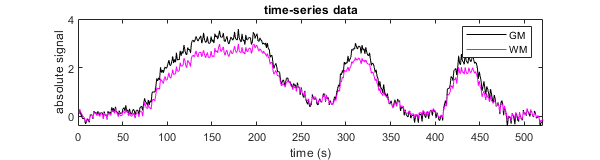clear TS1 TS2 nBOLD

### 4) Load end-tidal gas traces and temporally align with MRI data

For this purpose you canuse scripts located in the seeVR/gas_import/ folder (these are 'work in progress'). Typically, cross correlation between the gas trace of interest and a whole brain or gray-matter signal is used for initial bulk alignment. This approach is prone to errors due to diffrences in the expired gas trace vs. the hemodynamic response, as well as noise or other artefacts can influence the correlation. For this reason, seeVR includes an alignment tool. If you are using your own loading scripts for the end-tidal traces ensure that they are first resampled to the TR of your MRI data. This can be done using the 'resampletoTR' function included in the gas_import folder.
% Load breathing data based on RespirAct system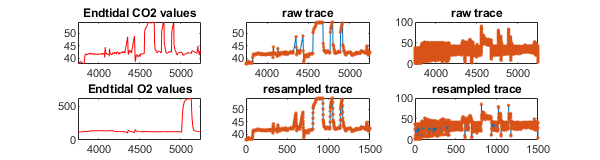% Use the GM time-series for alignment
% Run GUI to correct alignment. If you dont have O2 data, you can supply
% only the CO2 data. Correlation with the time-series is done using the first input.
trAlign(CO2_corr, O2_corr, TS, opts); % for this example the alignment offset was 7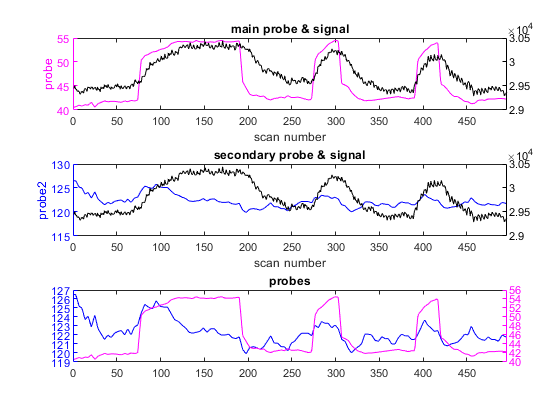% If both CO2 & O2 are supplied, the GUI returns two probe vectors to the
% Matlab workspace. If only CO2 or O2 are provided, then 1 probe is
% returned.
CO2trace = probe1;
O2trace = probe2;
clear TS probe1 probe2

### 5) Regress out motion parameters

To regress out motion parameters (or whatever other nuisance data you can think of), use the 'scrubData' function. This function accepts an array or nuisance regressors and data regressors. For the data probe we will use the end-tidal CO2 trace. You can also consider to first convolve the CO2 trace with an HRF using the ' convHRF' or ' convEXP' functions (see manual). Sometimes motion parameters are correlated with your task - this can be due to real motion (i.e. head movement during breath-hold) or effects related to changing signal intensity due to increased CBF that are confused as motion by the realignment algorithm. If including such regressors you risk removing signals of interest. To filter these you can use the ' filtRegressor' function.
cd(dir) % Go to our data directory
mpfilename = 'BOLD_mcf.par'; % Find MCFLIRT motion parameter file
% Calculate motion derivatives
dtnuisance = gradient(nuisance); % temporal derivative
sqnuisance = nuisance.*nuisance; % square of motion
motion = [nuisance dtnuisance sqnuisance];
% Demean/rescale regressors
for ii=1:(size(motion,2)); motion(:,ii) = demeanData(rescale(motion(:,ii))); end
% Filter regressors
opts.motioncorr = 0.3; % correlation (r) threshold
[keep, leave] = filtRegressor(motion, reference, opts);
% Attempt to clean all voxels contained in the WB mask using nuisance
% regressors. In this example, there are not many motion related artifacts.
opts.disp = [5 15 25 35]; %dispersion
[~,~,HRF_probe_CO2] = convHRF(CO2trace, opts);
%regression
% add a linear term to acount for signal drift
% Data scrubbing
[cleanData] = scrubData(BOLD,WBmask, keep, [HRF_probe_CO2], opts);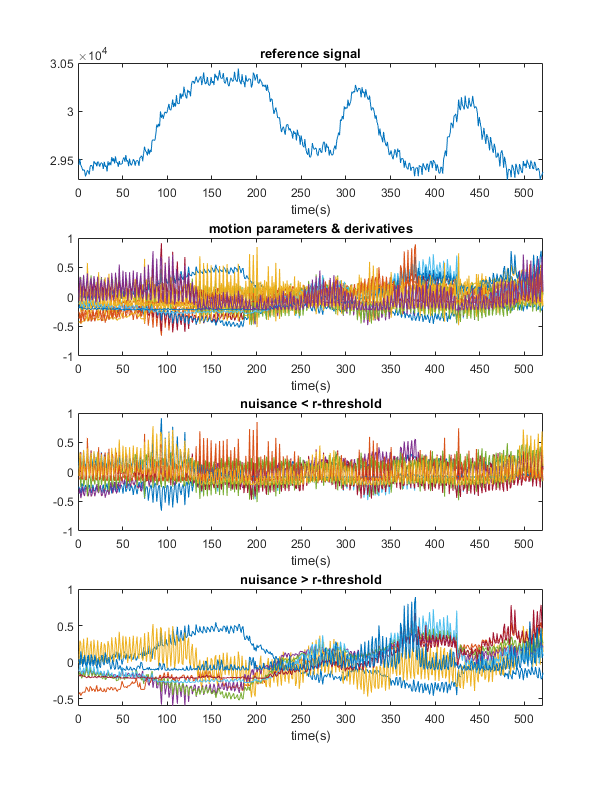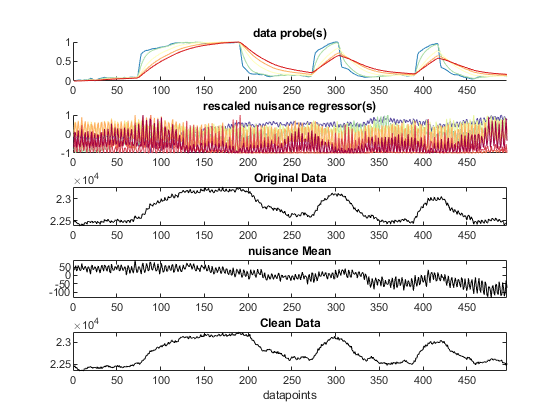% You can also consider to include the nuisance probes with high
% correlation to the reference signal as data probes or a linear term
%L = rescale(LegendreN(1,xdata));
%[cleanData] = scrubData(BOLD,WBmask, [L' keep], [HRF_probe_CO2' leave], opts);
% plot results
figure;
hold on
xlabel('time (s)'); ylabel('absolute signal');
title('whole-brain average signal')
legend('before cleanup', 'after cleanup');
set(gcf, 'Units', 'pixels', 'Position', [200, 500, 600, 160]);
xlim([0 xdata(end)]); hold off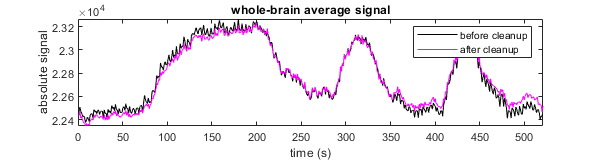### 6) Isolate data of interest

For this example we will focus on the first two hypercapnic blocks. We can reduce the data by using the 'chopTimeseries' function. The opts.headers.ts, and opts.dyn variables will also be updated to reflect the reduced number of volumes if they are already present in the opts structure. The opts.header.ts is also updated in case data is to be saved.
% Similar to the normTimeseries function, chopTimeseries can be supplied
% with a 2 element vector [idx1 idx2]. If no indicies are provided, manual
% input will be requested (try it to isolate different data)
[idx, rBOLD] = chopTimeseries(cleanData, GMmask, [1 390]);
%establish new xdata vector for plotting
xdata = opts.TR:opts.TR:opts.TR*size(rBOLD,4);
% IMPORTANT use the index values to isolate the corresponding CO2/O2 values
PetCO2 = CO2trace(1,idx(1):idx(2));
PetO2 = O2trace(1,idx(1):idx(2));

### 7) Removing contributions using a large vessel mask

CVR data can often be weighted by contributions from large vessels that may overshadow signals of interest. Particulary, for very high resolution acquisitions at high field strength. We can modify the whole brain mask to exclude CSF and large vessel contributions using the 'remLV' function ('remove large vessels'). This can be done by specifying the percentile threshold from which to remove voxels, or if the appropriate toolbox is not available, a manual threshold value.
% Supply necessary options
% Define the cutoff percentile (higher values are removed; default = 98);
opts.LVpercentile = 97;
% If the stats toolbox is not present, then a manual threshold must
% be applied. This can vary depending on the data (i.e. trial and error).
%plot results
figure;
z = 40;
subplot(1,3,1)
imagesc(imrotate(mean(BOLD(:,:,z,:),4),90));
title('mean image', 'Color', 'w'); colormap(gray); cleanPlot;
subplot(1,3,2)
subplot(1,3,3)
set(gcf, 'Units', 'pixels', 'Position', [200, 500, 600, 160]);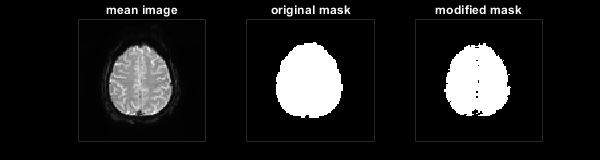### 8) Temporally de-noise data

To remove spikes from motion or high frequency noise these try the 'denoiseData' function. When the wavelet toolbox is available, this function uses a wavelet-based approach. Otherwise a more simple gaussian approach is applied however this can result in a temporal shift. Low-pass filtering is another option - this can be done via output from the 'glmCVRidx' function (see manual).
%de-noise
% Check results
figure; hold on;
title('noise removal');
ylabel('absolute signal'); xlabel('time (s)'); legend('original', 'de-noised')
set(gcf, 'Units', 'pixels', 'Position', [200, 500, 600, 160]); xlim([0 xdata(end)])
hold off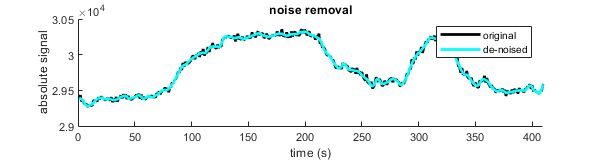clear cleanData

### 9) Smooth and normalize data

Here we will use some of the default parameters from the 'smthData' function. This means we do not need to pass any parameters in the opts struct (defaults are: opts.FWHM = 4mm, opts.filtWidth = 5)
% 3d Gaussian smoothing
opts.spatialdim = 3; %default = 2
Finished smoothing Data
% Normalize data to first 15 baseline images
clear sBOLD denBOLD

### 10) Calculate CVR and hemodynamic lag

Finally on to the fun stuff. The lagCVR function takes the PetCO2 input (or PetO2) and returns CVR maps and hemodynamic lag maps along with several statistical maps for data evaluation. This function has many options that can all impact your results. I've tried to set some basic defaults that should work with a variety of input data. For more information see the manual or explore the code and just try stuff.
% lagCVR has the option to add a nuissance regressor (for example a PetCO2
% trace when focusing on using PetO2 as the main explanatory regressor).
% This regressor is only used when opts.glm_model = 1 (default = 0). For
% now we won't include anything.
L = [];
% Setup some extra options - most are default but we will initialized them
% for the sake of this tutorial (see manual)
% We would like to generate CVR maps
opts.cvr_maps = 1; %default is 1
% Factor by which to temporally interpolate data. Better for picking up
% lags between TR. Higher value means longer processing time and more RAM
% used (so be careful)
opts.interp_factor = 2; %default is 4
% The correlation threshold is an important parameter for generating the
% optimized regressor. For noisy data, a too low value will throw an error.
% Ideally this should be set as high as possible, but may need some trial
% and error.
opts.corrthresh = 0.7; %default is 0.7
% Thresholds for refinening optimized regressors. If you make this range too large
% it smears out your regressor and lag resolution is lost. When using a CO2
% probe, the initial 'bulk' alignment becomes important here as well. A bad
% alignment will mean this range is also maybe not appropriate or should be
% widened for best results. (ASSUMING TR ~1s!, careful).
opts.lowerlagthresh = -2; %default is -3
opts.upperlagthresh = 2; %default is 3
% Lag thresholds (in units of TR) for lag map creation. Since we are looking at a healthy
% brain, this can be limited to between 20-60TRs. For impariment you can consider
% to raise the opper threshold to between 60-90TRs (ASSUMING TR ~1s!, careful).
opts.lowlag = -3; %setup lower lag limit; negative for misalignment and noisy correlation
opts.highlag = 25; %setups upper lag limit; allow for long lags associated with pathology
% For comparison we can also run the lagged GLM analysis (beware this can
% take quite some time)
opts.glm_model = 1; %default is 0
opts.corr_model = 1; %default is 1
% If you already have a probe from a previous run (with the correct
% length), you can opts.load_probe = 1 and the existing probe will be
% loaded - probe optimization will then be skipped
opts.load_probe = 0; %default is 0
% Produces the optimized regressor (default = 1) using the RAPIDTIDE approach.
% When this is set to 0; a straight correlation with the input probe is
% done.
% For creating the optimized BOLD regressor, we will only use GM voxels for
% maximum CO2 sensitivity. We will also remove large vessel contributions.
%remove CSF from analysis
%Perform hemodyamic analysis
% The lagCVR function saves all maps and also returns them in a struct for
% further analysis. It also returns the optimized probe when applicable.
probename = 'final_probe.mat'
Creating optimized regressor Correlating TS voxelwise to probe 1 . . . finished correlation, estimating new probe
rmse = 2.1757e+03
Correlating TS voxelwise to probe 2 . . . finished correlation, estimating new probe
rmse = 0.0789
Correlating TS voxelwise to probe 3 . . . finished correlation, estimating new probe
rmse = 0.0295
Correlating TS voxelwise to probe 4 . . . finished correlation, estimating new probe
rmse = 0.0011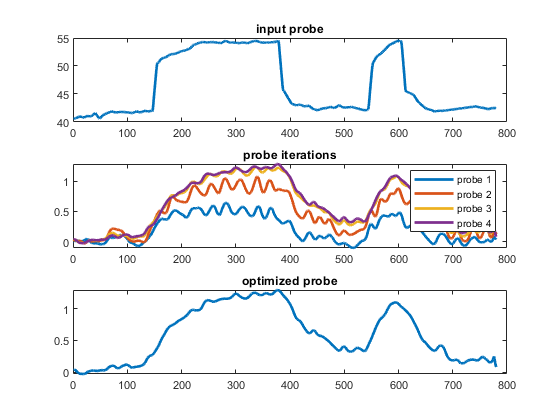Finished creating optimized regressor performing correlation based lag analysis using optimized regressor performing correlation based lag analysis using input probe Lag, regressor and r_maps were created in: 3 minutes Generating base maps using probe trace Base CVR, r2, Tstat and SSE maps were created using entidal regressor Generating lag-adjusted maps Stimulus response data is saved performing GLM based lag analysis using OPTIMIZED regressor Generating lag-adjusted CVR maps based on GLM analysis performing GLM based lag analysis using INPUT regressor Generating lag-adjusted CVR maps based on GLM analysis finished running GLM analysis in: 8 minutes saving maps in .mat file
% The advantage of using the global opts struct is that the variables used
% for a particular processing run (including all defaults set within
% functions themselves) can be saved to compare between runs.
save([opts.resultsdir,'processing_options.mat'], 'opts');

### 11) Plot results

% Plot resulting lag map with CVR maps
lag_xcorr = maps.XCORR.lag_opti; lag_xcorr(lag_xcorr == 0) = NaN;
r_xcorr = maps.XCORR.r_opti; r_xcorr(r_xcorr == 0) = NaN;
cvr_xcorr = maps.XCORR.CVR.bCVR; cvr_xcorr(cvr_xcorr == 0) = NaN;
ccvr_xcorr = maps.XCORR.CVR.cCVR; ccvr_xcorr(ccvr_xcorr == 0) = NaN;
lag_glm = maps.GLM.optiReg_lags; lag_glm(lag_glm == 0) = NaN;
r2_glm = maps.GLM.optiReg_R2; r2_glm(r2_glm == 0) = NaN;
cvr_glm = maps.GLM.CVR.optiReg_cCVR; cvr_glm(cvr_glm == 0) = NaN;
figure;
step = 5;
z=24;
scl = [0 0.35];
scllag = [0 20];
sclr = [-1 1];
r2 = [0 1];
for ii=0:step-1
% BOLD
subplot(5,5,ii+1)
imagesc(imrotate(BOLD(:,:,z + ii*step,1),90));
colormap(gray)
freezeColors;
cleanPlot; if ii == 0; ylabel('BOLD', 'color', 'w'); end
% Lags cross-correlation
subplot(5,5,ii+1+step)
imagesc(imrotate(lag_xcorr(:,:,z + ii*step),90), scllag);
colormap(magma)
freezeColors;
cleanPlot; if ii == 0; ylabel('lags xcorr', 'color', 'w');
xlabel('lags: 0 to 20s', 'color', 'w'); end
% Lags shifted regressor GLM
subplot(5,5,(ii+1+2*step))
imagesc(imrotate(lag_glm(:,:,z + ii*step),90), scllag);
colormap(magma)
freezeColors;
cleanPlot; if ii == 0; ylabel('lags glm', 'color', 'w');
xlabel('lags: 0 to 20s', 'color', 'w'); end
subplot(5,5,(ii+1+3*step))
imagesc(imrotate(r_xcorr(:,:,z + ii*step),90), sclr);
colormap(devon)
freezeColors;
cleanPlot; if ii == 0; ylabel('r xcorr', 'color', 'w');
xlabel('r: -1 to 1', 'color', 'w'); end
subplot(5,5,(ii+1+4*step))
imagesc(imrotate(r2_glm(:,:,z + ii*step),90), r2);
colormap(devon)
freezeColors;
cleanPlot; if ii == 0; ylabel('r2 glm', 'color', 'w');
xlabel('r2: 0 to 1', 'color', 'w'); end
end
set(gcf, 'Units', 'pixels', 'Position', [10, 100, 1000, 800]);